Processing ......FreeComputerBooks.com Links to Free Computer, Mathematics, Technical Books all over the World

A Concise Introduction to Mathematical Logic
GIS Visualizer - Geographic Data Visualized on 40+ Maps! Click here for details.
• Title: A Concise Introduction to Mathematical Logic
• Author(s) Wolfgang Rautenberg
• Publisher: Springer; 3rd ed. edition (December 17, 2009)
• Paperback: 340 pages
• eBook: PDF
• Language: English
• ISBN-10: 1441912207
• ISBN-13: 978-1441912206 (Print) 978-1441912213 (Online)Book Description

The textbook by Professor Wolfgang Rautenberg is a well-written introduction to the beautiful and coherent subject of mathematical logic. It contains classical material such as logical calculi, beginnings of model theory, and Goedel's incompleteness theorems, as well as some topics motivated by applications, such as a chapter on logic programming.

This is essentially the English translation of the third edition of the German version [Einführung in die mathematische Logik. Ein Lehrbuch. Wiesbaden: Vieweg+Teubner (2008; Zb1 1152.03-002)] of this well-written textbook. The book remains one of the most recommendable introductions into mathematical logic for mathematicians, and well-suited for computer scientists too.

This book treats the most important material in a concise and streamlined fashion. The third edition is a thorough and expanded revision of the former. Although the book is intended for use as a graduate text, the first three chapters can easily be read by undergraduates interested in mathematical logic.

• Wolfgang Rautenberg was a German mathematician and logician whose areas of research were model theory, non-classical logic, modal logic, temporal logic and self reference.
Reviews, Ratings, and Recommendations: Related Book Categories: Read and Download Links:Similar Books:
•Introduction to Mathematical Logic (Vilnis Detlovs, et al)

This book explores the principal topics of mathematical logic. It covers propositional logic, first-order logic, first-order number theory, axiomatic set theory, and the theory of computability. Discusses the major results of Gödel, Church, Kleene, Rosser, and Turing.

•A Friendly Introduction to Mathematical Logic (Chris Leary)

In this user-friendly book, readers with no previous study in the field are introduced to the basics of model theory, proof theory, and computability theory, leading to rigorous proofs of Gödel's First and Second Incompleteness Theorems.

•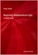Beginning Mathematical Logic: A Study Guide (Peter Smith)

It introduces the core topics and recommends the best books for studying these topics enjoyably and effectively. An invaluable resource both for those wanting to teach themselves new areas of logic and for those looking for supplementary reading.

•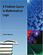A Problem Course in Mathematical Logic (Stefan Bilaniuk)

It is intended to serve as the text for an introduction to mathematical logic for undergraduates with some mathematical sophistication. It supplies definitions, statements of results, and problems, along with some explanations, examples, and hints.

•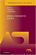Model-Theoretic Logics (Jon Barwise, et al)

This book brings together several directions of work in model theory between the late 1950s and early 1980s. It provides an introduction to the subject as a whole, as well as to the basic theory and examples. Many chapters can be read independently.

•Language, Proof and Logic (Jon Barwise, et al)

This book covers first-order language in a method appropriate for first and second courses in logic, and is specially useful to undergraduates of philosophy, computer science, mathematics, and linguistics.

•Logical Reasoning (Bradley H. Dowden)

The goal of this book is to improve your logical-reasoning skills. Your most important critical thinking skill is your skill at making judgments-not snap judgments that occur in the blink of an eye, but those that require careful reasoning.

•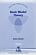Basic Model Theory (Kees Doets)

As the title indicates, this book introduces the reader to what is basic in model theory. A special feature is its use of the Ehrenfeucht game by which the reader is familiarised with the world of models.

•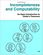Incompleteness and Computability: Gödel's Theorems

This book is an introduction to metamathematics and Gödel's Theorems. It covers recursive function theory, arithmetization of syntax, the first and second incompleteness theorem, models of arithmetic, second-order logic, and the lambda calculus.

Book Categories
 :All CategoriesTop BooksRecent BooksMiscellaneous BooksComputer LanguagesComputer ScienceData Science/DatabasesElectrical EngineeringJava and Java EE (J2EE)Linux and UnixMathematicsMicrosoft and .NETMobile ComputingNetworking and CommunicationsSoftware EngineeringSpecial TopicsWeb Programming
Other Categories